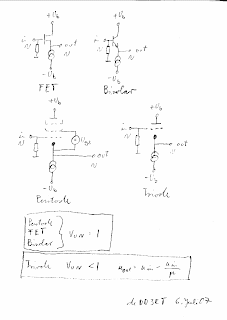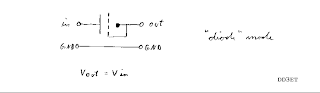## Monday, August 20, 2007

### Coupling Triodes

The fourth circuit topology for triodesHello,
I want to show you a new valve design. The greatest advantage of solid state circuits over valve designs is the complementary circuit topology.
Current mirrors make it possible to build up DC-coupled transistor amplifiers. There is no need for coupling elements like transformers, capacitors, resistor dividers, VDRs or neon bulbs, floating supplies etc. OpAmps as we know today are based on complementary circuit topology. Solid state circuit designers know the advantages of this design technique. A good solid state design has a balanced amount of NPN and PNP transistors. Now imagine what happens if you are allowed to use NPN types only. This is what valve circuit designers have to deal with, there are only "NPN valves" available. This gives a big restriction in valve circuit design. The design niveau of valve circuits today is the same as the early transistor designs. This is one reason why some people make hybrids, i.e. using transistors to upgrade valve circuits.
The special characteristic of a triode (!) makes it possible to break free from this restriction and get into a new world of valve circuit design.

Searching for a DC coupled valve amp I came up to the following idea:The red line shows the signal path. Note that there are only triodes in the signal path.
Three fundamental topologies are generally known. These are grounded cathode, grounded grid and grounded plate=(i.e. cathode follower).
A further circuit topology is possible with triodes only. In this basic circuit the anode is the input and the cathode the output.This makes a lot of new circuits possible. One of them is the RIAA triode (triode coupling) , which finds practical application for the first time in the RIAA 2007. But first I will show you some special aspects in the characteristics of the three well known basic circuits using triodes.
Cathode follower: In the picture you can see various cathode, emitter and source followers.The triode follower differs from the other configutations in that it has a gain less than one, even without load and with a current source. More specifically said the gain is reduced by the portion of the input voltage divided by µ. If one wants to keep this loss small, a tube with high µ has to be used. Each current change in the cathode follower generates slope distortions. These do not arise if the current in the tube remains constant. Thus the cathode follower should work in a no-load operation.

Grounded cathode and grounded grid circuit: The triode works here without mutal conductance slope distortions if its current remains constant. Unfortunalely, today this aspect receives little attention therefor I would like to deal here with it in more detail.
If one compares today's circuits with older ones one will see that today triodes are driven with a substantially higher current than in former times. One tries to get on a straight part of the graph to get low mutal conductance slope destortions. Remaining slope distortions are minimized with negative feedback. This makes special demands which from a typical low frequency triode like the ECC83 are only partially fulfilled however better by RF valves. These (e.g. ECC85) are often seen in sound amplifiers now.
We do not have to use triodes this way. Mutal conductance slope distortions are not an issue if anode current is held constant. One way to achieve this is to operate the triode with no-load and thus to work µ-gain. To get this operating condition the triodes internal resistance should be much smaller than the external load resistor. This operating condition can be reached easily using triodes with low µ like the ECC82 µ=17 but for high µ triodes like the ECC83 µ=100 we must think about a solution.
It would be possible to achieve this for high µ triodes by puting several in parallel without changing the voltage ratio. In this way the gain will increase to µ and the mutal conductance slope distortions will tend to zero. Alternatively it is possible to increase the external load and to reduce the current at the same time. By this means the voltage ratios are maintained.
The table on S.144 in the Valvo manual 1962-63 for ECC83 illustrates this:

Operating voltage 250V, output voltage 6V RMS, AC load = 3xRa
Ra=47K Ia=1,95mA voltage gain amount =36 distortion K=5.6%
Ra=100K Ia=1,23mA voltage gain amount =48 distortion K=5.1%
Ra=220K Ia=0,67mA voltage gain amount =57 distortion K=4.4%

In each of this configutationes the gain is still far from µ. If the signal voltage is small there is no need to drop half of the operating voltage at the anode. This makes it possible to increase the working resistance and the voltage gain as well as improving linearity. By this methode the triode can be operated linearly without applying negative feedback and this is the way that I have designed the RIAA 2007.
Enough now about the three well-known basic topologies.
In the fourth basic topology the anode is the input and the cathode is the output. For this basic circuit there are again different modes of operation, which permit a number of new circuit designs.
First a small signal view of these modes:
The grid is AC grounded. In this mode the input signal voltage at the anode appears at the cathode divided by the factor µ. Also the input impedance is divided by the factor µ.

Pay regards to the marginal conditions: The AC load impedance at the cathode should be a much higher impedance than the reciprocal value of the triode mutal conductance. If there is no change of anode current there is no slope distortion. The internal resistance of the triode does not have noticeable influence in the transformer mode. In this mode the triode can be used for example for low impedance output coupling.
If the grid is small signal connected to the cathode, no more transformation takes place. In this mode of operation diode mode mentioned,the input voltage is passed through from the anode to the cathode. Compared to the transformer mode, diode mode differs in that the complete internal resistance of the triode is in series to the signal. The AC load impedance at the cathode needs to be much higher than the triode's internal resistance. If there is no change of anode current there is no slope distortion and in the diode mode a signal can be coupled to different DC level without loss. In the e.g. STC topology negative feedback from anode to grid of the tetrode is made by a triode in the diode mode.

The vari µ modemakes all signal division ratios between 1 and µ possible. This mode can be used in driving OTL (=series push pull) stages.

In the filter modea frequency transfer function can be made. This mode is used in the RIAA triode. It’ll be explained later in the text.

Large signal view for the fourth basic topology:
A DC anode current generates a voltage drop between anode and cathode. This voltage drop can be adjusted by a biasing voltage at the grid of the triode. Thus the triode is able to couple signals between stages with different DC potentials. Triodes in this mode of operation are called coupling triodes. For the first time they make it possible to build real DC coupled valve amplifiers with no DC potential between input and output. There is no need for coupling elements like transformers, capacitors, resistor dividers, VDRs or neons, floating supplies etc. used in former times. In solid state designs this DC coupling is made with complementary current mirrors.

RIAA 2007 blog

Updated Feb. 27. 2009